受限玻尔兹曼机(RBM)+对比散度算法(CD-k)

• 主要内容：
• 受限玻尔兹曼机（RBM）基本原理
• 受限玻尔兹曼机（RBM）训练过程——对比散度算法（CD-k）

1. 受限玻尔兹曼机（RBM）基本原理
受限玻尔兹曼机(RBM)是一种典型的神经网络模型，由一层可视层 v$v$ 和一层隐藏层 h$h$ 组成，该网络的可视层 v$v$ 和隐藏层 h$h$ 神经元彼此互联，但同一层内神经元无连接，如图1。RBM能够通过隐藏层获得可视层神经元的高阶相关性，因此可以通过RBM进行特征提取。RBM中神经元有两种状态:“激活”和“未激活”，一般用二进制的1和0表示。受限玻尔兹曼机一个最主要的优点是在给定可视层神经元状态时，隐藏层某一神经元的状态是条件独立于该隐藏层其它神经元的状态；反之，各个可视层神经元的状态亦条件独立。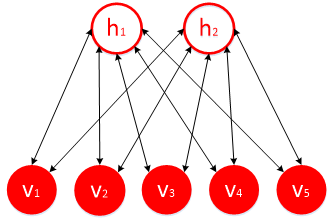受限玻尔兹曼机是一种基于能量的模型，可视层神经元向量 v$v$ 和隐藏层神经元向量 h$h$ 联合配置的能量函数为：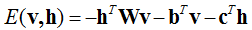(11)$(1-1)$

联合似然为：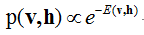(12)$(1-2)$

条件似然函数为：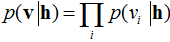(13)$(1-3)$

隐藏层各神经元的条件概率为：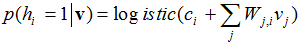(14)$(1-4)$

可视层各神经元的条件概率为：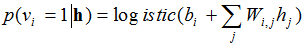(15)$(1-5)$

若 b$b$c$c$W$W$ 均为训练好的参数。对于样本集中任意一个样本点 xi=(xi,1,xi,2,...,xi,n)$x_{i}= ( x_{i,1},x_{i,2},...,x_{i,n} )$ (n为属性个数)，其属性值 xi,j$x_{i,j}$ 对应可视层神经元 vj$v_{j}$。根据公式(1-4)，将样本点 xi$x_{i}$ 所有属性值代入公式(1-4)，可得特征提取后的样本点 xi$x_{i}^{'}$
接下来介绍如何对RBM进行训练得到参数 b$b$c$c$W$W$

2. 受限玻尔兹曼机（RBM）训练过程——对比散度算法（CD-k）
学习RBM的任务是求出参数的值，来拟合给定的训练数据。Hinton提出了RBM的一个快速学习算法，即对比散度(Contrastive Divergence，CD)。由于CD-k算法中（k表示采样次数），当k=1时，即只进行一步吉布斯采样，就能达到很好的拟合效果。故一般采用CD-1算法的形式，来拟合各参数的值，如图2。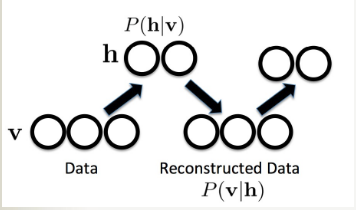设可视层 v$v$ 的重构（reconstruction）为 v$v^{*}$，根据重构的可视层 v$v^{*}$ 所得隐藏层为 h$h^{*}$ 。设学习效率为 ε$\varepsilon$，经过对比散度算法对RBM进行训练后，权重矩阵 W$W$ 、可视层的偏置向量 b$b$ 、隐藏层的偏置向量 c$c$，根据文献，更新规则如下：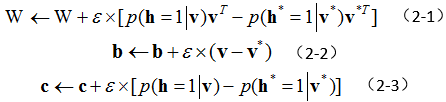RBM的对比散度算法主要步骤：根据训练集中样本点设置可视层神经元 v$v$ 激活状态，根据公式（1-4）计算所有隐藏层神经元状态；在隐藏层各神经元的状态 h$h$ 确定之后，根据公式（1-5）计算在隐藏层确定的情况下，所有可视层神经元的状态，从而产生可视层的一个重构 v$v^{*}$ ；如果此时 v$v$v$v^{*}$ 一样，那么得到的隐藏层 h$h$ 就是可视层 v$v$ 的另外一种表达，此时隐藏层可以作为可视层输入数据的特征。同时在训练中，可以利用可视层的状态与重构可视层的状态的误差来调整RBM的参数，从而使得 v$v$v$v^{*}$ 的重构误差尽可能减小。

对于初学者来讲，在训练RBM的过程中，并不了解如何根据样本点 xi=(xi,1,xi,2,...,xi,n)$x_{i}= ( x_{i,1},x_{i,2},...,x_{i,n} )$ (n为属性个数)来设置对应可视层 v$v$ 的状态，以及如何设置隐藏层神经元的状态。此处提供一种常用的方法，并做简要说明：
对于训练集 S$S$ 中所有样本点 (x1,x2,...,xN)$( x_{1},x_{2},...,x_{N} )$（N为训练样本点的个数），计算在第 j$j$ 个属性下所有样本点的最大值 maxiN(xi,j)$\underset{i\in N}{max}(x_{i,j})$，以及最小值miniN(xi,j)$\underset{i\in N}{min}(x_{i,j})$，当xi,jminiN(xi,j)maxiN(xi,j)miniN(xi,j)$\frac{x_{i,j}-\underset{i\in N}{min}(x_{i,j})}{\underset{i\in N}{max}(x_{i,j})-\underset{i\in N}{min}(x_{i,j})}$ 大于阈值 δj,1$\delta _{j,1}$ 时，则对应可视层神经元状态处于激活状态，即“1”，否则为“0”。将可视层神经元状态值，此时只有“0”和“1”，代入至公式（1-4），得到隐藏层神经元的激活概率，当激活概率大于阈值 δj,2$\delta _{j,2}$ 时，隐藏层神经元的状态值为“1”，否则为“0”。阈值 δj,1$\delta _{j,1}$δj,2$\delta _{j,2}$ 的设定，可以根据数据集的不同有所不同，亦或是随机产生[0,1]上的随机数，作为 δj,1$\delta _{j,1}$δj,2$\delta _{j,2}$ 的值。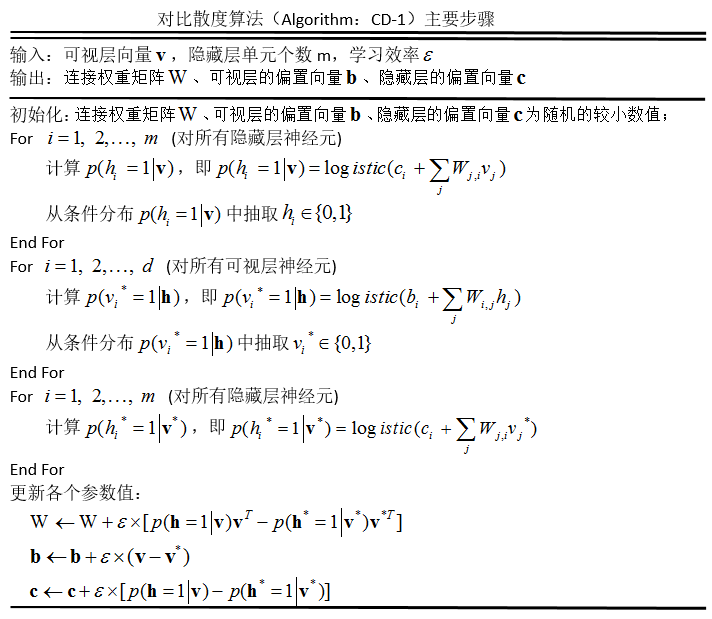关于对比散度算法中，相关参数的设置，文献给出了一些初步的方法，感兴趣的小童鞋，可以了解一下。

• 参考文献
 Hinton G E. Training products of experts by minimizing contrastive divergence[J]. Neural Computation, 2002, 14(8):1771-1800.
 Bengio Y. Learning Deep Architectures for AI[J]. Foundations & Trends® in Machine Learning, 2009, 2(1):1-127.
 张春霞, 姬楠楠, 王冠伟. 受限波尔兹曼机?[J]. 工程数学学报, 2015(2):159-173.